# Plotting data distributions with Swift Charts

In this post I'm going to explore how to visualise data distributions with Swift Charts framework.

I want to look at a dataset of some survey data collected from penguin colonies. The dataset captures many different anatomical details, such as weight, flipper length and bill length. To start my investigation, I will look at the distribution of values for each of these parameters.

To build a histogram, I need to group my data into bins and count how many samples are within each bin. Charts provides a simple way to do the binning operation with NumberBins data types.

The `NumberBins` datatype has a range of constructors. The simplest one to use is init(data:desiredCount:), it detects the best placement of bin thresholds from the input data.

``````let bins = NumberBins(
data: dataset.map(\.billLength),
desiredCount: 30
)
``````

However, just using `NumberBins` is not enough, it doesn't group the data into bins. It just provides a way to easily determine which bin each bit of data belongs to. To group the data I need to use Dictionary.init(grouping:by:) constructor along with the bins to group the data.

``````let groups = Dictionary(
grouping: dataset.map(\.billLength),
by: bins.index
)
``````

Now I have a dictionary that maps the bin index to an array of all the values in that bin.

To plot a histogram, I need to compute the proportion of all the data samples that are in each bin.

``````let preparedData = groups.map { key, values in
(
index: key,
range: bins[key],
frequency: values.count
)
}
``````

I'm computing the proportion of samples and, at the same time, retrieving the range of values that correspond to this bin.

Since the number of samples is small enough, I will just place all of the above work into a computed property.

``````struct PenguinChart: View {
let dataset: [PenguinsDataPoint]

var binnedData: [
(
index: Int,
range: ChartBinRange<Float>,
frequency: Int
)
] {
let bins = NumberBins(
data: dataset.map(\.billLength),
desiredCount: 30
)

let groups = Dictionary(
grouping: dataset.map(\.billLength),
by: bins.index
)

let preparedData = groups.map { key, values in
return (
index: key,
range: bins[key],
frequency: values.count
)
}

return preparedData
}

var body: some View { ... }
}
``````

With the data aggregated into bins, it's time to plot a bar chart.

``````struct PenguinChart: View {
let dataset: [PenguinsDataPoint]

var binnedData: [(...)} { ... }

var body: some View {
Chart(
self.binnedData, id: \.index
) { element in
BarMark(
x: .value(
"Bill Length",
element.range
),
y: .value(
"Frequency",
element.frequency
)
)
}
}
}
``````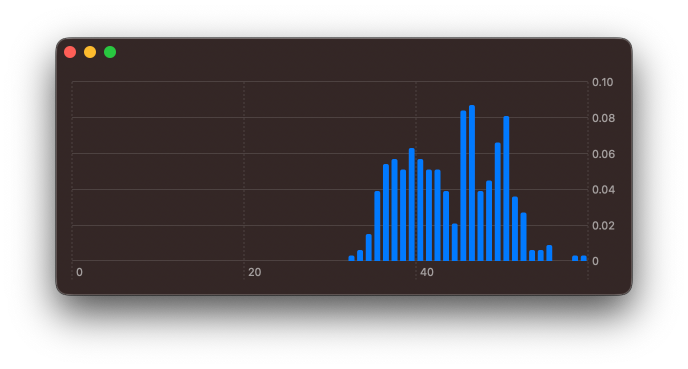The chart looks a little off, since the framework always forces the axes to start at `0` by default. Using chartXScale(domain: .automatic(includesZero: false)) modifier I can override the default here.

``````struct PenguinChart: View {
let dataset: [PenguinsDataPoint]

var binnedData: [(...)} { ... }

var body: some View {
Chart(self.binnedData, id: \.index) { element in
BarMark(
x: .value(
"Bill Length",
element.range
),
y: .value(
"Frequency",
element.frequency
)
)
}
.chartXScale(
domain: .automatic(includesZero: false)
)
}
}
``````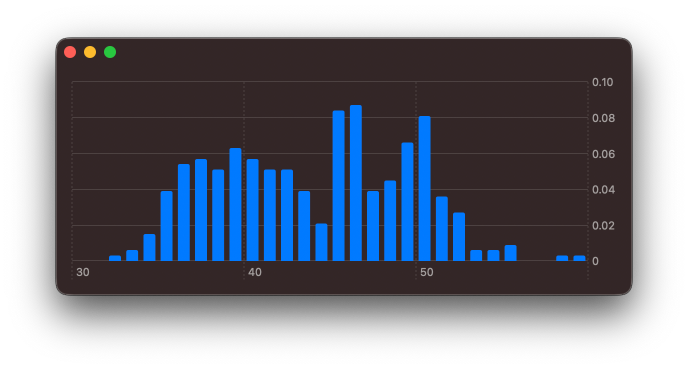Let's explore the other numerical properties of the data set. I can adjust the computed `binnedData` to aggregate over other fields of the dataset.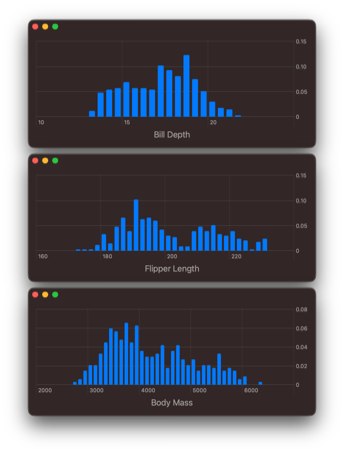Plotting just one attribute at a time is useful, however, I would love to drill down on the relationship between these variables.

## # Building a 2D density plot

I feel it would be best to look into the relationship between flipper length and bill length, as both of these appear to be multimodal distributions.

I need to adjust how I'm aggregating the data. To start with, I will create bins for both flipper length and bill length.

``````let flipperLengthBins = NumberBins(
data: dataset.map(\.flipperLength),
desiredCount: 30
)

let billLengthBins = NumberBins(
data: dataset.map(\.billLength),
desiredCount: 30
)
``````

To represent each grid square, I will create an intermediate data type, that combines a `flipperLengthBin` index and a `billLengthBin` index.

``````struct TwoDimensionalBinIndex: Hashable {
let xBinIndex: Int
let yBinIndex: Int
}
``````

`TwoDimensionalBinIndex` must conform to `Hashable`, so that it can be used with `Dictionary.init(grouping:by:)`. When grouping the data, I will create the corresponding `TwoDimensionalBinIndex` for each value.

``````let groupedData = Dictionary(
grouping: dataset
) { element in
TwoDimensionalBinIndex(
xBinIndex:
flipperLengthBins
.index(for: element.flipperLength),
yBinIndex:
billLengthBins
.index(for: element.billLength)
)
}
``````

I'm calling index(for:) method of the `NumberBin` instances for each element in my data set and assigning that bin index to the corresponding `x` and `y` attributes in the `TwoDimensionalBinIndex`, that is used to group the data.

As with the regular histogram, I now need to aggregate these values for each group. This time, rather than returning just a single range, I will need to return a range for `x` and a range for `y`.

``````let values = groupedData
.map { key, values in
return (
index: key,
xDataRange: flipperLengthBins[
key.xBinIndex
],
yDataRange: billLengthBins[
key.yBinIndex
],
frequency: values.count
)
}
``````

Here subscript(position:) method on the respective `NumberBins` instances is used to retrieve the ChartBinRange values for each axes. To bring this all together, it's best to first declare a `typealias` for the aggregated binned values.

``````typealias BinnedValue = (
index: TwoDimensionalBinIndex,
xDataRange: ChartBinRange<Float>,
yDataRange: ChartBinRange<Float>,
frequency: Int
)
``````

In my chart view, as with the histogram, I use a regular computed property.

``````struct PenguinChart: View {
let dataSet: [PenguinsDataPoint]

struct TwoDimensionalBinIndex: Hashable {
let xBinIndex: Int
let yBinIndex: Int
}

typealias BinnedValue = (
index: TwoDimensionalBinIndex,
xDataRange: ChartBinRange<Float>,
yDataRange: ChartBinRange<Float>,
frequency: Int
)

var bins: [BinnedValue] {
let flipperLengthBins = NumberBins(
data: dataset.map(\.flipperLength),
desiredCount: 25
)

let billLengthBins = NumberBins(
data: dataset.map(\.billLength),
desiredCount: 25
)

let groupedData = Dictionary(
grouping: dataset
) { element in
TwoDimensionalBinIndex(
xBinIndex: flipperLengthBins
.index(for: element.flipperLength),
yBinIndex: billLengthBins
.index(for: element.billLength)
)
}

let values = groupedData
.map { key, values in
return (
index: key,
xDataRange: flipperLengthBins[
key.xBinIndex
],
yDataRange: billLengthBins[
key.yBinIndex
],
frequency: values.count
)
}

return values
}

var body: some View { ... }
}
``````

With all these bins computed, it's now a matter of plotting the values.

``````struct PenguinChart: View {
let dataSet: [PenguinsDataPoint]

...

var bins: [BinnedValue] { .. }

var body: some View {
Chart(
self.bins, id: \.index
) { element in
RectangleMark(
x: .value(
"Flipper Length",
element.xDataRange
),
y: .value(
"Bill Length",
element.yDataRange
)
)
.foregroundStyle(
by: .value(
"Frequency",
element.frequency
)
)
}
.chartXScale(
domain: .automatic(
includesZero: false
)
)
.chartYScale(
domain: .automatic(
includesZero: false
)
)
}
}
``````

I use a RectangleMark to draw a rectangle for each `x` and `y` region, then I colour that region based on the frequency value. Furthermore, as with the histogram, I adjust the `x` and `y` axes to no longer start at `0`.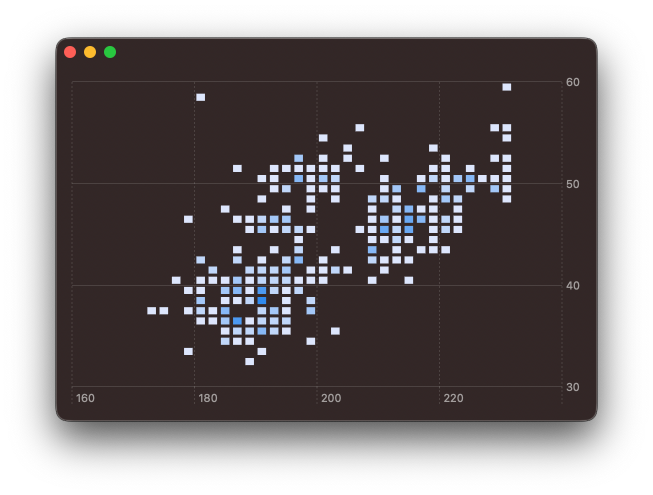Clearly, some styling is going to be needed. I will adjust the colour gradient and get rid of the spacing between each of the rectangles. The colour gradient can be adjusted using chartForegroundStyleScale(range:) modifier. And by passing width and height values to `RectangleMark` initialiser, I'm able to controle the spacing provided.

``````struct PenguinChart: View {

...

var body: some View {
Chart(
self.bins, id: \.index
) { element in
RectangleMark(
x: .value(
"Flipper Length",
element.xDataRange
),
y: .value(
"Bill Length",
element.yDataRange
),
width: .ratio(1),
height: .ratio(1)
)
.foregroundStyle(
by: .value(
"Frequency",
element.frequency
)
)
}
.chartXScale(
domain: .automatic(
includesZero: false
)
)
.chartYScale(
domain: .automatic(
includesZero: false
)
)
.chartForegroundStyleScale(
colors: [
Color.red.opacity(0.1),
Color.yellow
]
)
)
}
}
``````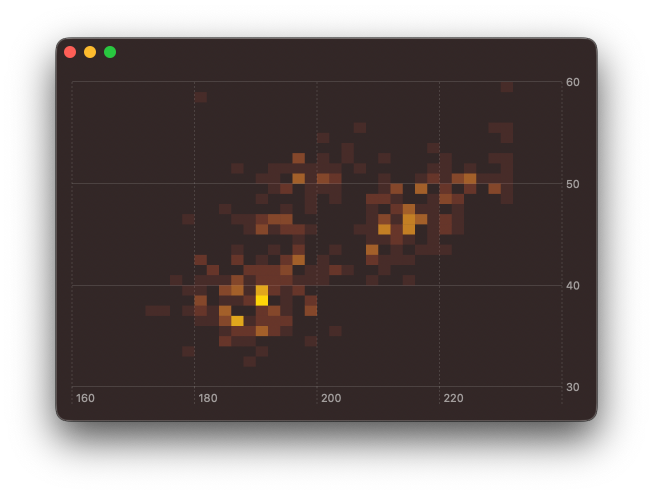While exploring this, I did also tried using `BarMark` instead of or `RectangleMark` by varying the width and height and I was able to create some interesting effects. These could be very useful if animating between different plotted attributes, but that will need to wait for a follow up post.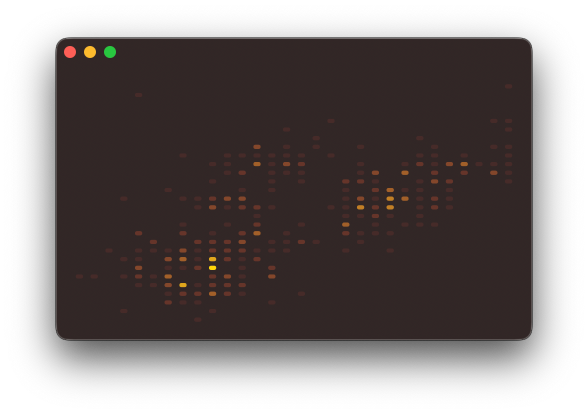You can find the code for this post in our GitHub project, this includes the code to download and parse the CSV file.